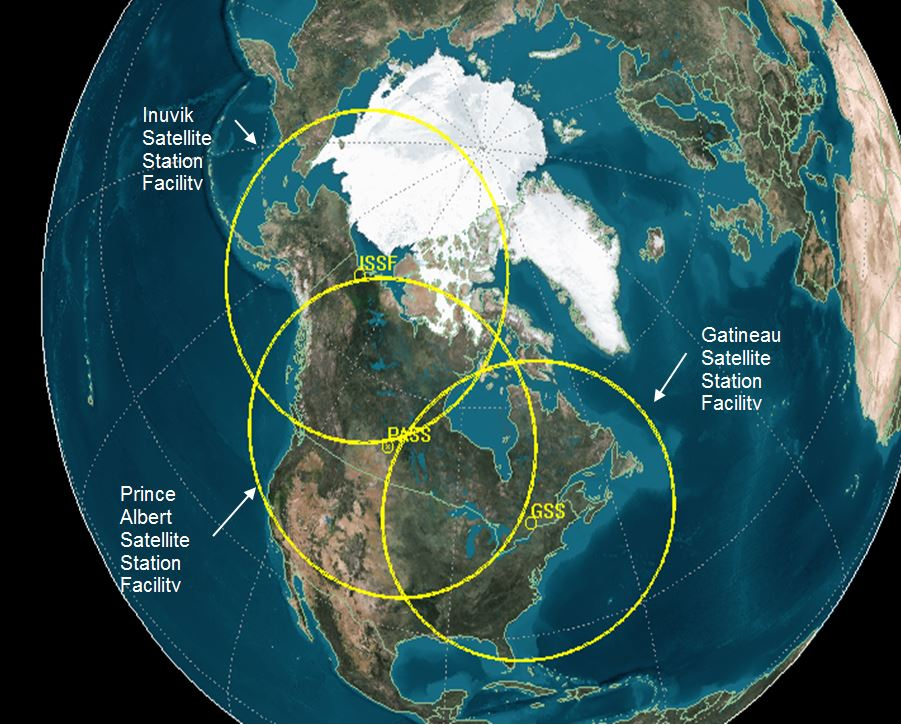# Satellite Coverage Area Calcs: AGI, LOCKHEED MARTIN AND NORTHROP GRUMMAN

AGI, LOCKHEED MARTIN AND NORTHROP GRUMMAN WERE INTERESTED IN A SOLUTION TO A SATELLITE COVERAGE PROBLEM. WE PLACED THE SOLUTION ONLINE TO HELP PEOPLE WITH THEIR ENGINEERING! THANKS!the problem is basically: what distance on the earth would represent the coverage of a satellite placed in orbit around the earth at a fixed altitude?

How would this problem differ in the case of mars?solution in python:

#THIS ROUTINE WILL CALCULATE COVERAGE DISTANCE ON THE EARTH BASED ON A SATELLITE IN ORBIT AT A GIVEN ALT

#THESE LINES COMPUTE THE ORBITAL RADIUS VECTOR OF THE SATELLITE IN ORBIT AROUND THE EARTH

r_earth = 12756.1433
satellite_alt = 800
r_vect = satellite_alt+r_earth

#THESE LINES COMPUTE THE VIEWING ANGLE FROM THE SAT

arc_sat = r_vect/r_earth
arc_sat
arc_sat_deg = 180*arc_sat/3.14
arc_sat_deg

#THESE LINES COMPUTE THE PROJECTED ANGLE IN THE EARTHS FRAME

arc_sat_distance = arc_sat_deg * (3.14/180)*r_vect

angle_earth = arc_sat_distance / r_earth

angle_earth_deg = 180*(angle_earth)/3.14

angle_earth_deg

#NOTE THAT THE RESULT DIFFERS BY A SMALL DELTA

#THESE LINES COMPUTE THE DISTANCE ON THE EARTH THAT IS VISIBLE TO THE SAT

dist_earth = angle_earth*r_earth
dist_earth

This site uses Akismet to reduce spam. Learn how your comment data is processed.